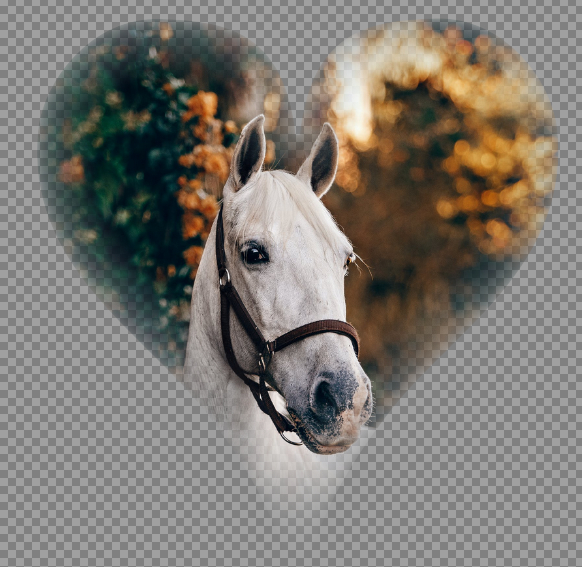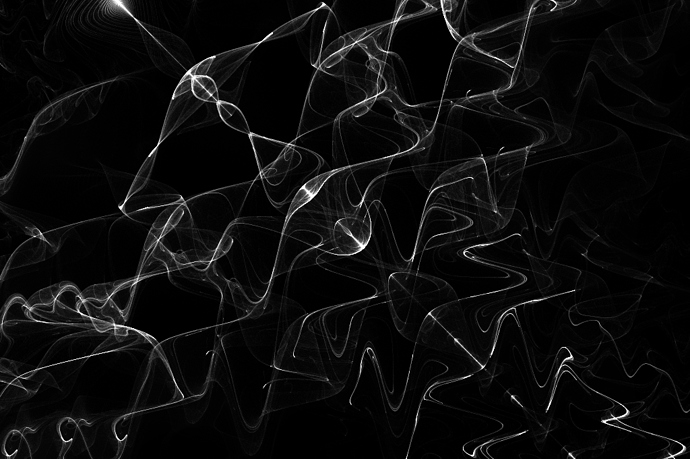# Reptillian

Members

566

4

## Posts posted by Reptillian

1. ### What music are you listening to?

I'll just leave this here.

2. ### Finding millions of colors that does or does not exist - G'MIC QT Tutorial

Update on this tutorial: Because of the G'MIC-QT update provided by null54, you can use this filter on a existing image, then paste into a new image provided the original image is smaller than 4096^2.

•1
3. ### Reptorian's G'MIC Code Workshop

New Filter - Bit Plane Shuffler. For PDN users, just use 8-bit by default as that PDN is limited to.

4. ### how do I make opacity higher than it already is?

On 5/22/2020 at 12:09 PM, BoltBait said:

At 255 your opacity is set to completely solid... for every other software on the planet.

What about softwares that allows 16-bit images? RGBA16I is a thing.

As for the OP, I think this might be your problem. 8-bit depth limitation in PDN.

5. ### border around an image

What do you mean by zigzag? The 90 angle edges?

If it is:

Either increase image resolution or switch to a vector graphics software. It's a inherent limitation of raster graphics.

6. ### Reptorian's G'MIC Code Workshop

Added some more changes to Edge Fade. It now respect the boundary of distance. Meaning your image will not disappear.

•3
7. ### Reptorian's G'MIC Code Workshop

Sorry for the slow filter! I have pushed a faster version utilizing squared-euclidean distance calculation. Now it finish in 3 seconds on my machine. If it slow and takes 3+ minutes, then it haven't been updated. It even looks much nicer too!

8. ### OldPC - MSX & ZX Spectrum graphic emulator

EDIT: Found it, it's under Adjustment->Colors.

9. ### Reptorian's G'MIC Code Workshop

For anyone that tried the Edge fade filter, is it too slow? It would finish in less than a minute on a 420x420 image on my own machine. The algorithm currently use upscaling for increased precision.

10. ### Reptorian's G'MIC Code WorkshopReleased new filter called Edge Fade. It is similar to PDN plugin named Edge Fader Extreme. However, my version is a more precise filter with the option of localized normalization preprocessing step. That means it can work in dusty alphas. It is more precise as it use a circular eroding pattern, and subpixel processing.

Photo is from Helena at Pexels.

•2
•2
11. ### Remove Selected (Row/Column) + Join/Splice Together

3 hours ago, dbareis said:

Thanks for the responses, I had to rebuild my computer hence the late reply.  None of those options seems easier than just swapping to a different program (and I almost always use the zigzag effect).  If it annoys me enough I'll investigate writing a plugin to do it.

That actually brings me a idea for PDN. @Rick Brewster What do you think of adding Erase Blending Mode? Instead of adding alpha, it does the exact opposite using the blend layer.

12. ### Reptorian's G'MIC Code Workshop

Here's what I have coded in. Paste it into code filter under G'MIC-QT->Various section and see what you get. You get this image:```rep_pfrac 100,1,.25,10,1,0,0,0,1,0,0,1
rep_pfrac: rep_popcorn_fractal \$*
#\$1==points_per_pixels#
#\$2==density#
#\$3==H#
#\$4==K#
#\$5==zoom#
#\$6==rotation_angle#
#\$7==origin_x#
#\$8==origin_y#
#\$9==formulamode#
rep_popcorn_fractal:
skip \${1=50},\${2=1},\${3=.05},\${4=3},\${5=1},\${6=0},\${7=0},\${8=0},\${9=0},\${10=},\${11=},\${12=},\${13=},\${14=},\${15=}
f 0 r 100%,100%,1,1
repeat \$! l[\$>]
eval \${-math_lib}"
const pts=\$1;
const density=1/\$2;
const H=\$3;
const K=\$4;
const zoom=1/\$5;
const ang=(\$6/180)*pi;
const origin_x=\$7*-1*zoom;
const origin_y=\$8*zoom;
const sd=max(w,h)/min(w,h);
const sx=w>h?sd:1;
const sy=w>h?1:sd;
const cx=w/2;
const cy=h/2;
const ocx=origin_x*cx;
const ocy=origin_y*cy;
const angcondition=(\$6-360*floor(\$6/360))?1;
rot_x(a,b)=a*cos(ang)-b*sin(ang);
rot_y(a,b)=a*sin(ang)+b*cos(ang);
if(!\$9,
if(narg(\$10),
if((\$10%3)==0,func_a(a)=sin(a);,
if((\$10%3)==1,func_a(a)=cos(a);,
if((\$10%3)==2,func_a(a)=tan(a);
);
);
);,
func_a(a)=sin(a);
);
if(narg(\$11),
if((\$11%3)==0,func_b(a)=sin(a);,
if((\$11%3)==1,func_b(a)=cos(a);,
if((\$11%3)==2,func_b(a)=tan(a);
);
);
);,
func_b(a)=tan(a);
);
if(!narg(\$12),
if(narg(\$10),
if((\$10%3)==0,func_c(a)=sin(a);,
if((\$10%3)==1,func_c(a)=cos(a);,
if((\$10%3)==2,func_c(a)=tan(a);
);
);
);,
func_c(a)=sin(a);
);,
if((\$12%3)==0,func_c(a)=sin(a);,
if((\$12%3)==1,func_c(a)=cos(a);,
if((\$12%3)==2,func_c(a)=tan(a);
);
);
);
);
if(!narg(\$13),
if(narg(\$11),
if((\$11%3)==0,func_d(a)=sin(a);,
if((\$11%3)==1,func_d(a)=cos(a);,
if((\$11%3)==2,func_d(a)=tan(a);
);
);
);,
func_d(a)=tan(a);
);,
if((\$13%3)==0,func_d(a)=sin(a);,
if((\$13%3)==1,func_d(a)=cos(a);,
if((\$13%3)==2,func_d(a)=tan(a);
);
);
);
);
popcorn_x(a,b)=a-H*func_a(b+func_b(K*b));
popcorn_y(a,b)=b-H*func_c(a+func_d(K*a));
,
if(narg(\$10),
if((\$10%3)==0,func_a(a)=sin(a);,
if((\$10%3)==1,func_a(a)=cos(a);,
if((\$10%3)==2,func_a(a)=tan(a);
);
);
);,
func_a(a)=sin(a);
);
if(narg(\$11),
if((\$11%3)==0,func_b(a)=sin(a);,
if((\$11%3)==1,func_b(a)=cos(a);,
if((\$11%3)==2,func_b(a)=tan(a);
);
);
);,
func_b(a)=cos(a);
);
if(narg(\$12),
if((\$12%3)==0,func_c(a)=sin(a);,
if((\$12%3)==1,func_c(a)=cos(a);,
if((\$12%3)==2,func_c(a)=tan(a);
);
);
);,
func_c(a)=tan(a);
);
if(!narg(\$13),
if(narg(\$10),
if((\$10%3)==0,func_d(a)=sin(a);,
if((\$10%3)==1,func_d(a)=cos(a);,
if((\$10%3)==2,func_d(a)=tan(a);
);
);
);,
func_d(a)=sin(a);
);,
if((\$13%3)==0,func_d(a)=sin(a);,
if((\$13%3)==1,func_d(a)=cos(a);,
if((\$13%3)==2,func_d(a)=tan(a);
);
);
);
);
if(!narg(\$14),
if(narg(\$11),
if((\$11%3)==0,func_e(a)=sin(a);,
if((\$11%3)==1,func_e(a)=cos(a);,
if((\$11%3)==2,func_e(a)=tan(a);
);
);
);,
func_e(a)=cos(a);
);,
if((\$14%3)==0,func_e(a)=sin(a);,
if((\$14%3)==1,func_e(a)=cos(a);,
if((\$14%3)==2,func_e(a)=tan(a);
);
);
);
);
if(!narg(\$15),
if(narg(\$12),
if((\$12%3)==0,func_f(a)=sin(a);,
if((\$12%3)==1,func_f(a)=cos(a);,
if((\$12%3)==2,func_f(a)=tan(a);
);
);
);,
func_f(a)=tan(a);
);,
if((\$12%3)==0,func_f(a)=sin(a);,
if((\$12%3)==1,func_f(a)=cos(a);,
if((\$12%3)==2,func_f(a)=tan(a);
);
);
);
);
popcorn_x(a,b)=a-H*func_a(b+func_b(K*b+func_c(K*b)));
popcorn_y(a,b)=b-H*func_a(a+func_b(K*a+func_c(K*a)));
);
for(ix=0,ix<w,ix+=density,
for(iy=0,iy<h,iy+=density,
xx=zoom*(ix-cx)/cx;
yy=zoom*(iy-cy)/cy;
xx*=sx;
yy*=sy;
xx+=origin_x;
yy+=origin_y;
for(ptn=0,ptn<pts,ptn++,
xnew=popcorn_x(xx,yy);
ynew=popcorn_y(xx,yy);
xval=((xnew-origin_x*sx)*cx/zoom+cx*sx)/sx;
yval=((ynew-origin_y*sy)*cy/zoom+cy*sy)/sy;
if(angcondition,
temp_xval=xval-cx+ocx;
temp_yval=yval-cy+ocy;
xval=rot_x(temp_xval,temp_yval)+cx-ocx;
yval=rot_y(temp_xval,temp_yval)+cy-ocy;
);
if((round(xval)>=0&&round(yval)>=0)&&(round(xval)<w&&round(yval)<h),
i(#0,round(xval),round(yval),0,0)+=1;
);
xx=xnew;
yy=ynew;
);
);
);
"
endl done```

•1
13. ### Reptorian's G'MIC Code Workshop

I got news. Where I'm at, hospitals are getting filled with COVID-19 patients and in fact, less than 10 counties have hospitals at 10- percent capacity. Actually, I do not think it would be wise to try to find a job when I already have a roof over me. So, I will code up a few things.

•3
14. ### [Question] Plugin development - custom window rather than effect?

If there has to be extension to what PDN plugin supports, I would like to see plugins being able to access multiple layers. I may be biased as a inactive gmic-qt scripter that enjoys being able to work with multiple layers within GIMP/Krita. That would solve the group layer issue indirectly by temporarily treating layers as group layer, and enable batch filtering.

15. ### Outline Object

On 6/28/2020 at 4:07 AM, Foxxey said:

I've been using this plugin for so long and it's amazing. The only thing that bothers me is this bug:

If im trying to create a outline with a Softness of 209 for example its creating this weird "alpha value jumps"

255-235-188-141-94-47-0:

Its a very minor bug and can be fixed (kinda) with a quick gaussian blur..

That may have to do with softness setting. I'm not sure if this is even a bug at all. How I would fix that is to use lerp(a,b,c) to allow users to set up a transition between soft and hard.

16. ### Wanted: optical flow in paint filter

Have a look at gmic-qt plugin and see artistic section.

•1
17. ### What music are you listening to?

Could honestly listen to this all day.

•3
18. ### Reptorian's G'MIC Code Workshop

3 hours ago, Seerose said:

❤️ Dear

Gmic collection. zip, I opened it, I don't know where the folder is.

That .zip file is a collection of note in gmic code form. It's only for scripters and programmers. Some of them can be copied and pasted into Custom Code gui filter.

If you really want to code within g'mic scripting, I would start with downloading KDE Kate and open those files with KDE Kate (That is what I use), look into Custom Code filters under Various under gmic-qt plugin under paint.net. Then, use the g'mic reference, and read tutorials on how to code. In a way, it's a lot easier to code in filters with gmic-qt than it is to work with c# or c++.  Another source you can use to learn it is to read G'MIC-QT STDLIB or look at the g'mic-community repository. Almost every codes I done for g'mic-qt is found within reptorian.gmic. If you need more help, I would ask discuss.pixls.us forum, and they'll help you understand how everything works better than I can.

•1
19. ### CodeLab v6.0 (for advanced users) Released June 25, 2020

Back when I used CodeLab, I actually did struggled with autocomplete at times. Most of the time, I would like to have it disabled as it is a struggle at times. I don't look at keyboard when typing in.

20. ### Reptorian's G'MIC Code Workshop

As I have mentioned I had plans to leave some files, well and TODO list. Here it is. I also did one final update with major reformatting.

Here's some of the TODOs.

And, then here's some of my note files. - GMIC collection.zip

Thank you for your support. @Seerose @lynxster4 @Eli @Djisves and other people here.

•2
21. ### G'MIC (06-15-2020)

24 minutes ago, Pixey said:

Ahhh - so it's rather like TR's morpher, which produces multiple layers, which then have to be uploaded to PDN to make it animated.

It would be nice to do the same in G'MIC tooThanks.

Well, it turns out that the solution I have proposed isn't desirable, so, only @null54 can address this.

@null54 : If you'd like to address this issue, please look at this thread for more discussion on this. - https://discuss.pixls.us/t/gmic-morph-interactive-paint-net-workaround/18749

22. ### G'MIC (06-15-2020)

9 minutes ago, Pixey said:

Hi @Reptillian the OP seemed to suggest that they had managed to use the Morph[interactive] but that they did not know how to save it?  If they got that far, it seems to suggest they got it to work.  A mystery to me.

Saving it implies multiple output pictures. On Krita and GIMP, when you work with g'mic-qt filters that generates multiple layers from the interface, you get outputs of multiple layers. That is not possible on Paint.NET. Hence, why I was contacting one of the main maintainer of g'mic-qt to add a option to allow exports into a folder into Morph [Interactive], so @zaffer can save the outputs.

23. ### 'Deformations / Morph [Interactive]'

@zaffer I should let you know that I am notifying @G'MIC to add a workaround for Paint.NET users, so you can generate multiple pictures and output to a folder. So, one wouldn't need to use TR's Morpher.

24. ### G'MIC (06-15-2020)

@Pixey As far as I'm concerned, Paint.NET can only output to the same layer a plugin applies the effect on. So, this means that the filter needs workaround support akin to filters I have made workarounds for Paint.NET such as Non-Isometric Tiler and Tiled Form. I will notify @G'MIC as he is the author of the Morph[Interactive] filter. He can edit the current filter, and please notify the author of another thread asking for help on Morph that I have notified @G'MIC about this issue. So, it would be possible to generate multiple pictures generated from the Morph [Interactive] and output into a folder once this gets addressed.

25. ### Reptorian's G'MIC Code Workshop

I got news, guys! I decided to get a job (couldn't earlier because of fatigue issues, and coronavirus lockdowns), so I will not be making filters for g'mic-qt any longer. There is a upgrade to Tiled Form filter though.

In interest of programmers here - I will leave files and drop a note within Testing->Reptorian for anyone that wish to take over. This thread may be locked right after I do that though I may leave it open if any programmers asks me the intrinsic of those effects and how they work. I have not decided on that yet.

•2
•2